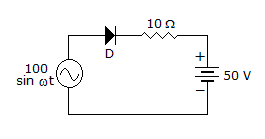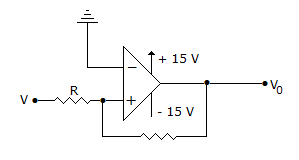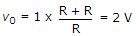# Electronics and Communication Engineering - Analog Electronics

26.

In the circuit of figure diode will conductA. from 90 to 180° in the positive half cycle B. from 30° to 180° in the positive half cycle C. from 50° to 160° in the positive half cycle D. from 30° to 150° in the positive half cycle

Explanation:

Diode will conduct when instantaneous value of ac voltage is more than 50 V.

27.

The input to integrating op-amp is a rectangular wave. The output will be

 A. a sine wave B. a rectangular wave C. a triangular wave D. none of the above

Explanation:

Integral of constant is t.

28.

The most widely used diode rectifier circuit is

 A. half wave B. full wave with centre tapped transformer C. bridge D. either (a) or (b)

Explanation:

Bridge rectifier has the advantage of lower PIV. Moreover it does not require centre tapped transformer.

29.

In figure, V0 =A. -1 V B. 2 V C. +1 V D. +15 V

Explanation:.

30.

The main advantage of a crystal oscillator

 A. can oscillate any frequency B. gives a high output voltage C. frequency of oscillation is almost constant D. can operates at a low dc voltage